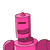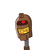# the difference between two numbers is ten and their sum is 4 time the smaller number. find the two numbers

the difference between two numbers is ten and their sum is 4 time the smaller number. find the two numbers

### 2 thoughts on “the difference between two numbers is ten and their sum is 4 time the smaller number. find the two numbers”

1.To Solve:

• Find the original two number.

Given:

• the difference between two numbers is ten.
• their sum is 4 time the smaller number.

Solⁿ:

• Let greater no. be = x

• Let smaller no. be = x

ATQ;

» x – y = 10 ———(1)

» x + y = 4y

x = 4y – y

= x = 3y

Substitute the value of x as 3y in eqⁿ 1.

3y – y = 10

2y = 10

y = 5

Value of x :

x – y = 10

x – 5 = 10

x = 10 + 5

x = 15

### ★The Numbers Are 5,15.

HOPE IT HELPS

MARK BRAINLIEST PLS 🙂

2.The numbers are 5 and 15

## Solution

Let the numbers=x,y

Given,

x-y=10 (here, you can see that y is the smaller number. otherwise the answer would be negative)

x+y=4y

Further simplification:

x = 3y

3y – y = 10

or, y=5

or, x=3y=15

Hope it helps!